Review for Second Exam        MAT 131, Fall 1998

The midterm will be held on Tuesday, November 17 at 8:30 pm at locations to be announced. Be sure to bring your Stony Brook ID card and your calculator.

This handout is to help you review and study for the exam. It includes several problems which are similar to those on the exam. These problems are not exactly the same as those on the exam, but they should give you an idea of the kinds of things you need to know. However, you should also do a large number of additional problems from the text. Do not merely limit yourself to the assigned problems or those given here. Solutions to these problems should appear on the web page later this week.

The exam will cover material through section 4.3 in the text, as well as §4.6 and §4.8.

• You should be able to take the derivative of just about any expression consisting of standard functions. In particular, you should know how to take the derivative of power functions, trigonometric functions, exponentials and logarithms, and inverse trigonmetric functions such as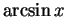and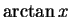. You also need to know how to apply the product, quotient, and chain rules to take derivatives of combinations of these functions.

• You need to understand the process of implicit differentiation-- how to calculate a derivative even when one cannot explicitly solve for y as a function of x.

• You should be able to simplify combinations of trigonmetric and inverse trigonometric functions, such as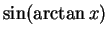. Do NOT memorize these formulae-- rather, understand how to calculate by drawing an appropriate triangle. You should also understand how to use inverse trigonometric functions in practice.

• You should be familiar with the idea of differential equations: how to translate between the symbolic language of DEs and English statements about rates of change, and how to check whether a function is a solution of a given differential equation. You do not need to know how to find solutions, although you should know that the solution to the equation y' = ay is of the form y(t) = k eat. When given a general solution to an initial value problem, you should be able to use the initial conditions to determine the appropriate value of the constants involved.

• You should be able to calculate the best linear approximation to a function at a point (the tangent line), and the best quadratic approximation. You should be able to use these to approximate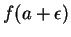when f(a), f'(a), and possibly f''(a) are known. You will not be asked questions on higher Taylor polynomials or the error bounds, although understanding these ideas will only help you.

• You should be able to do optimization word problems and related rates problems, determining from the English descriptions exactly what quantities are to be found and what the relevant equations are. You should not need to memorize any formulae, assuming you already know the Pythagorean theorem ( a2 + b2 = c2), how to compute the perimiter and area of a circle and a rectangle, and how to calculate the volume of a rectangular solid.

1. Compute the derivative with respect to x for each of the following expressions: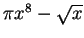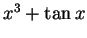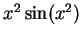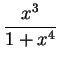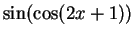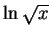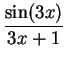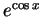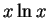2. Consider the elliptic curve C which consists of the set of points for which

x2 -x = y3 - y

(see the graph below).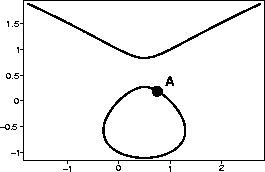a.
Write the equation of the line tangent to C at the point (1,0).
b.
Use your answer to part a to estimate the y-coordinate of the point with x-coordinate 3/4 marked A in the figure. Plug your estimate into the equation for C to determine how good it is.

c.
Write the equation of the parabola which best approximates C at the point (1,0).

d.
Use your answer to part c to improve your answer from part b. How close does this new estimate come to being right?

3. A mold culture is growing on the world's largest slice of bread. The culture starts in the center of the bread, and remains approximately circular.

a.
The size of the culture grows at a rate proportional to the square of its diameter. Write a differential equation which expresses this relationship.

b.
Verify that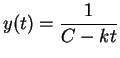satisfies the differential equation for any choice of k and C.

c.
If the diameter of the culture was 1 mm at 8 A.M. and 2 mm at noon, what is the size of the culture at 2 P.M.? What about at 3 P.M.? Does anything surprising happen at 4 P.M.?

4. A spotlight is aimed at a building whose base is 20 feet away. If the light is raised so that its angle increases at a constant rate of 5 degrees per second, how fast is the image rising when the light makes a 45 degree angle with the ground?

5. A poster is to be made which requires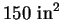for the printed part, and is to have a 3" margin at the top and bottom, and a 2" margin on the sides. What should the dimensions be in order to minimize the total area of the poster?

6. The stiffness of a beam is directly proportional to the product of its width and the cube of its breadth. What are the dimensions of the stiffest beam that can be cut from a cylindrical log with a radius of 2'?

7. At what x value does the maximum of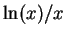occur? What is the maximum value of the function?

8. Compute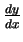for the curve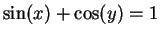. What is the slope of the tangent line at the point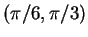?

Scott Sutherland
1998-11-11